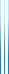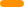# PublicationsPapers

## Lower bounds for context-free grammars

Yuval Filmus
Information Processing Letters, Volume 111, Issue 18, 2011, pp. 895–898

Ellul, Krawetz, Shallit and Wang prove an exponential lower bound on the size of any context-free grammar generating the language of all permutations over some alphabet. We generalize their method and obtain exponential lower bounds for many other languages, among them the set of all squares of given length, and the set of all words containing each symbol at most twice.

The version below corrects two typos in the proof of Proposition 6: $w_1 \sim w_2$ should be $x(w_1) \sim x(w_2)$, and in the following sentence $N^{-1}(A)$ should be $x(N^{-1}(A))$; and a typo in the statement of Theorem 9: the exponent should be $t$ rather than $n$.

## BibTeX

@article{Filmus2011,
author = {Yuval Filmus},
title = {Lower bounds for context-free grammars},
journal = {Information Processing Letters},
volume = {111},
issue = {18},
year = {2011},
pages = {895--898}
}copy to clipboard